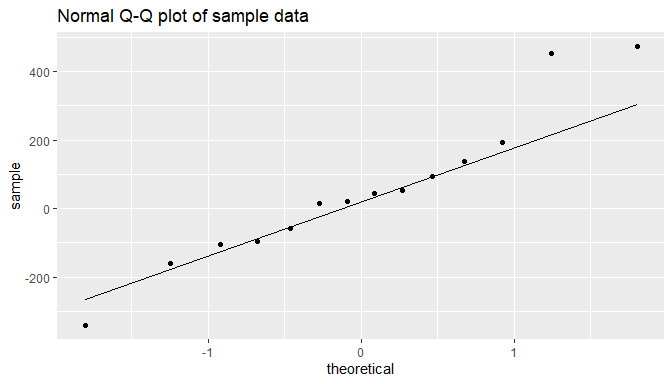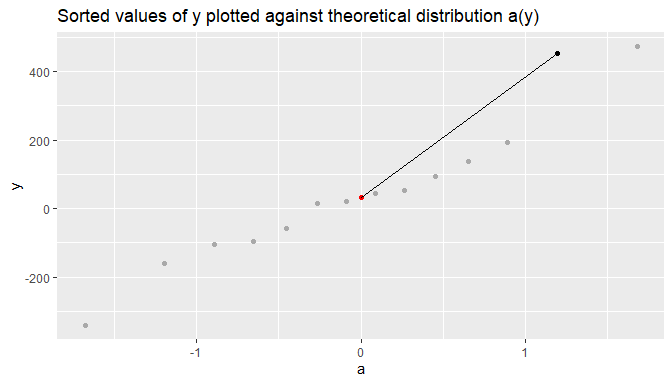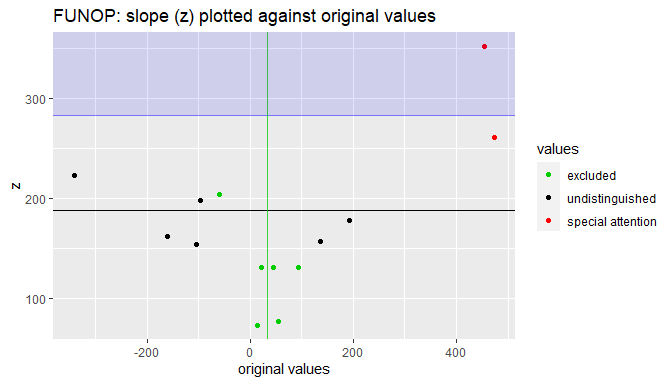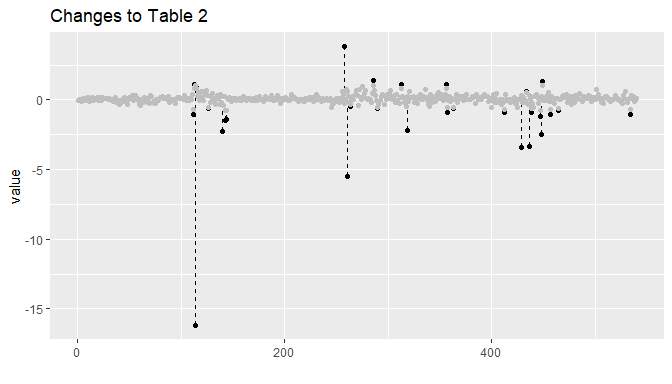# Vacuum Vignette

## A tidy implementation of John Tukey’s vacuum cleaner

One of John Tukey’s landmark papers, “The Future of Data Analysis” , contains a set of analytical procedures that focus on the analysis of two-way (contingency) tables. Vacuum contains an implementation of the three procedures:

• FUNOP (FUll NOrmal Plot): Identifies outliers within a vector by calculating the slope (z) of every element, relative to the vector’s median.

• FUNOR-FUNOM (FUll NOrmal Rejection-FUll NOrmal Modification): Treats a two-way (contingency) table for outliers by isolating residuals from the table’s likely systemic effects, which are calculated from the table’s grand, row, and column means.

• Vacuum cleaner: Removes systemic effects from values in a two-way table, returning a set of residuals that can be used for further examination (e.g., analysis of variance).

### FUNOP

The base prodecure is FUNOP, which identifies outliers in a vector. Here’s Tukey’s definition:

(b1) Let ai|n be a typical value for the ith ordered observation in a sample of n from a unit normal distribution.

(b2) Let y1y2 ≤ … ≤ yn be the ordered values to be examined. Let be their median (or let , read “y trimmed”, be the mean of the yi with n < i ≤ (2n).

(b3) For i ≤ ⅓n or > ⅓(2n) only, let zi = (yi)/ai|n (or let zi = (yi) /ai|n).

(b4) Let be the median of the z’s thus obtained (about (2n) in number).

(b5) Give special attention to z’s for which both |yi| ≥ A · and zi ≥ B · where A and B are prechosen.

(b5) Particularly for small n, zj’s with j* more extreme than an i for which (b5) selects zi also deserve special attention… (p 23).

The basic idea is very similar to a Q-Q plot.

Tukey gives us a sample of 14 data points. On a normal Q-Q plot, if data are normally distributed, they form a straight line. But in the chart below, based upon data from Tukey’s example, we can clearly see that a couple of the points are relatively distant from the straight line. They’re outliers.The goal of FUNOP, though, is to eliminate the need for visual inspection by automating interpretation.

The first variable in the FUNOP procedure (ai|n) simply gives us the theoretical distribution, where i is the ordinal position in the range 1..n and Gau-1 is the quantile function of the normal distribution (i.e., the “Q” in Q-Q plot):

$a_{i|n}=\ {\rm Gau}^{-1}\left[\frac{\left(3i-1\right)}{\left(3n+1\right)}\right]$

The key innovation of FUNOP is to calculate the slope of each point, relative to the median.If is the median of the sample, and we presume that it’s located at the midpoint of the distribution (where a(y) = 0), then the slope of each point can be calculated as:

$z_i=\frac{y_i - \acute{y}}{a_{i|n}}$

The chart above illustrates how slope of one point (1.2, 454) is calculated, relative to the median (0, 33.5).

$z\ =\ \frac{\Delta y}{\Delta a}\ =\ \frac{\left(454.0-\ 33.5\right)}{\left(1.2-0\right)}=350.4$

Any point that has a slope significantly steeper than the rest of the population is necessarily farther from the straight line. To do this, FUNOP simply compares each slope (zi) to the median of all calculated slopes ().

Note, however, that FUNOP calculates slopes for the top and bottom thirds of the sorted population only, in part because zi won’t vary much over the inner third, but also because the value of ai|n for the inner third will be close to 0 and dividing by ≈0 when calculating zi might lead to instability.

Significance—what Tukey calls “special attention”—is partially determined by B, one of two predetermined values (or hyperparameters). For his example, Tukey recommends a value between 1.5 and 2.0, which means that FUNOP simply checks whether the slope of any point, relative to the midpoint, is 1.5 or 2.0 times larger than the median.

The other predetermined value is A, which is roughly equivalent to the number of standard deviations of yi from and serves as a second criterion for significance.

The following chart shows how FUNOP works.Our original values are plotted along the x-axis. The points in the green make up the inner third of our sample, and we use them to calculate , the median of just those points, indicated by the green vertical line.

The points not in green make up the outer thirds (i.e., the top and bottom thirds) of our sample, and we use them to calculate , the median slope of just those points, indicated by the black horizontal line.

Our first selection criterion is ziB · . In his example, Tukey sets B = 1.5, so our threshold of interest is 1.5, indicated by the blue horizontal line. We’ll consider any point above that line (the shaded blue region) to “deserve special attention”. We have only one such point, colored red.

Our second criterion is |yi| ≥ A · . In his example, Tukey sets A = 0, so our threshold of interest is |yi| ≥ 0 or (more simply) yi. Basically, any point not on the green line. Our one point in the shaded blue region isn’t on the green line, so we still have our one point.

Our final criterion is any zj’s with j more extreme than any i selected so far. Basically, that’s any value more extreme than the ones already identified. In this case, we have one value that’s larger (further to the right on the x-axis) than our red dot. That point is also colored red, and we flag it as deserving special attention.

The two points identified by FUNOP are the same ones that we identified visually in Chart 1.

# Table 1 contains example data from Tukey's paper
table_1
#>     14 -104  -97  -59 -161   93  454 -341   54  137  473   45  193   22

result <- funop(table_1)
result
#>       y  i middle           a        z special
#> 1    14  6   TRUE -0.26540475       NA      NA
#> 2  -104  3  FALSE -0.89255967 154.0513   FALSE
#> 3   -97  4  FALSE -0.65630499 198.8405   FALSE
#> 4   -59  5   TRUE -0.45214741       NA      NA
#> 5  -161  2  FALSE -1.19379507 162.9258   FALSE
#> 6    93 10   TRUE  0.45214741       NA      NA
#> 7   454 13  FALSE  1.19379507 352.2380    TRUE
#> 8  -341  1  FALSE -1.67966119 222.9616   FALSE
#> 9    54  9   TRUE  0.26540475       NA      NA
#> 10  137 11  FALSE  0.65630499 157.7011   FALSE
#> 11  473 14  FALSE  1.67966119 261.6599    TRUE
#> 12   45  8   TRUE  0.08755225       NA      NA
#> 13  193 12  FALSE  0.89255967 178.6995   FALSE
#> 14   22  7   TRUE -0.08755225       NA      NA

# value of the outliers
result[which(resultspecial == TRUE), 'y'] #>  454 473 ### FUNOR-FUNOM A common reason for identifing outliers is to do something about them, often by trimming or Winsorizing the dataset. The former simply removes an equal number of values from upper and lower ends of a sorted dataset. Winsorizing is similar but doesn’t remove values. Instead, it replaces them with the closest original value not affected by the process. Tukey’s FUNOR-FUNOM offers an alternate approach. The procedure’s name reflects its purpose: FUNOR-FUNOM uses FUNOP to identify outliers, and then uses separate rejection and modification procedures to treat them. The technique offers a number of innovations. First, unlike trimming and Winsorizing, which affect all the values at the top and bottom ends of a sorted dataset, FUNOR-FUNOM uses FUNOP to identify individual outliers to treat. Second, FUNOR-FUNOM leverages statistical properties of the dataset to determine individual modifications for those outliers. FUNOR-FUNOM is specifically designed to operate on two-way (or contingency) tables. Similar to other techniques that operate on contingency tables, it uses the table’s grand mean (x..) and the row and column means (xj. and x.k, respectively) to calculate expected values for entries in the table. The equation below shows how these effects are combined. Because it’s unlikely for expected values to match the table’s actual values exactly, the equation includes a residual term (yjk) to account for any deviation. \begin{align} (actual \space value) &= (general \space \mathit{effect}) + (row \space \mathit{effect}) + (column \space \mathit{effect}) + (deviation)\\ x_{jk} &= (x_{..}) + (x_{j.} - x_{..}) + (x_{.k} - x_{..}) + (y_{jk})\\ &= x_{..} + x_{j.} - x_{..} + x_{.k} - x_{..} + y_{jk}\\ &= x_{j.} + x_{.k} - x_{..} + y_{jk} \end{align} FUNOR-FUNOM is primarily interested in the values that deviate most from their expected values, the ones with the largest residuals. So, to calculate residuals, simply swap the above equation around: $y_{jk}=x_{jk}-x_{j.}-\ x_{.k}+\ x_{..}\$ FUNOR-FUNOM starts by repeatedly applying FUNOP, looking for outlier residuals. When it finds them, it modifies the outlier with the greatest deviation by applying the following modification: $x_{jk}\rightarrow x_{jk}-{\Delta x}_{jk}$ where $\Delta x_{jk}=\ z_{jk} \cdot a_{a(n)}\cdot\frac{r\cdot c}{\left(r-1\right)\left(c-1\right)}$ Recalling the definition of slope (from FUNOP) $z_i=\frac{y_i - \acute{y}}{a_{i|n}}$ the first portion of the Δxjk equation reduces to just yjk, the difference of the residual from the median. The second portion of the equation is a factor, based solely upon table size, meant to compensate for the effect of an outlier on the table’s grand, row, and column means. When Δxjk is applied to the original value, the yjk terms cancel out, effectively setting the outlier to its expected value (based upon the combined effects of the contingency table) plus a factor of the median residual (~ xj. + x.k + x.. + ). FUNOR-FUNOM repeats this same process until it no longer finds values that “deserve special attention.” In the final phase, the FUNOM phase, the procedure uses a lower threshold of interest—FUNOP with a lower A—to identify a final set of outliers for treatment. The adjustment becomes $\Delta x_{jk} = (z_{jk} - B_M \cdot z̍)a_{i|n}$ There are a couple of changes here. First, the inclusion of (–BM · ) effectively sets the residual of outliers to FUNOP’s threshold of interest, much like the way that Winsorizing sets affected values to the same cut-off threshold. FUNOM, though, sets only the residual of affected values to that threshold: The greater part of the value is determined by the combined effects of the grand, row, and column means. Second, because we’ve already taken care of the largest outliers (whose adjustment would have a more significant effect on the table’s means), we no longer need the compensating factor. The chart below shows the result of applying FUNOR-FUNOM to the data in Table 2 of Tukey’s paper.The grey dots represent the cleansed dataset. The black dots represent the values of affected points prior to treatment, and the dashed lines connect those points to their treated values, illustrating the extent to which those points changed. FUNOR handles the largest adjustments, which Tukey accomplishes by setting AR = 10 and BR = 1.5 for that portion of the process, and FUNOP handles the finer adjustments by setting AM = 0 and BM = 1.5. Again, because the procedure leverages the statistical properties of the data, each of the resulting adjustments is unique. Here’s an example on a smaller dataset. # create a random dataset, representing a two-way table set.seed(42) dat <- matrix(rnorm(16), nrow = 4) # add some noise dat[2, 1] <- rnorm(1, mean = 10) # cleanse the table of outliers cleansed_dat <- funor_funom(dat) # look at the two tables, before and after dat #> [,1] [,2] [,3] [,4] #> [1,] 1.3709584 0.40426832 2.0184237 -1.3888607 #> [2,] 9.7157471 -0.10612452 -0.0627141 -0.2787888 #> [3,] 0.3631284 1.51152200 1.3048697 -0.1333213 #> [4,] 0.6328626 -0.09465904 2.2866454 0.6359504 cleansed_dat #> [,1] [,2] [,3] [,4] #> [1,] 1.3709584 0.40426832 2.0184237 -1.3888607 #> [2,] 9.2354713 -0.10612452 -0.0627141 -0.2787888 #> [3,] 0.3631284 1.51152200 1.3048697 -0.1333213 #> [4,] 0.6328626 -0.09465904 2.2866454 0.6359504 # look at the difference between the two tables # only one non-zero difference dat - cleansed_dat #> [,1] [,2] [,3] [,4] #> [1,] 0.0000000 0 0 0 #> [2,] 0.4802758 0 0 0 #> [3,] 0.0000000 0 0 0 #> [4,] 0.0000000 0 0 0 ### Vacuum cleaner FUNOR-FUNOM treats the outliers of a contingency table by identifying and minimizing outsized residuals, based upon the grand, row, and column means. Tukey takes these concepts further with his vacuum cleaner, whose output is a set of residuals, which can be used to better understand sources of variance in the data and enable more informed analysis. To isolate residuals, Tukey’s vacuum cleaner uses regression to break down the values from the contingency table into their constituent components (p 51): $\mathit{ (original \space values) = (dual \space regression) \\ + (deviations \space \mathit{of} \space row \space regression \space \mathit{from} \space dual \space regression) \\ + (deviations \space \mathit{of} \space column \space regression \space \mathit{from} \space dual \space regression) \\ + (residuals) \\ }$ The idea is very similar to the one based upon the grand, row, and column means. In fact, the first stage of the vacuum cleaner produces the same result as subtracting the combined effect of the means from the original values. To do this, the vacuum cleaner needs to calculate regression coefficients for each row and column based upon the values in our table (yrc) and a carrier—or regressor—for both rows (ar) and columns (bc). Below is the equation used to calculate regression coefficients for columns. $[y/a]_c= \frac{\sum_r{a_ry_{rc}}}{\sum_r a_r^2}$ Conveniently, the equation will give us the mean of a column when we set ar ≡ 1: $[y/a]_c= \frac{\sum_r{1 \cdot y_{rc}}}{\sum_r 1} = \frac{\sum_r{y_{rc}}}{n_r}$ where nr is the number of rows. Effectively, the equation iterates through every row (Σr), summing up the individual values in the same column (c) and dividing by the number of rows, the same as calculating the mean (y.c). Note, however, that ar is a vector. So to set ar ≡ 1, we need our vector to satisfy this equation: $\sum_r a_r^2=1$ For a vector of length nr we can simply assign every member the same value: $\sqrt{\frac{1}{n_r}}$ Our initial regressors end up being two sets of vectors, one for rows and one for columns, containing either √(1/nc) for rows or √(1/nr) for columns. Finally, in the same way that the mean of all row means or the mean of all column means can be used to calculate the grand mean, either the row coefficients or column coefficients can be used to calculate a dual-regression (or “grand”) coefficient: $\frac{\sum_{c}{\left[y/a\right]_cb_c}}{\sum_{c} b_c^2}\ \equiv\ \frac{\sum\sum{a_ry_{rc}b_c}}{\sum\sum{a_r^2b_c^2}}\equiv\frac{\sum_{r}{a_r\left[y/b\right]_r}}{\sum_{r} a_r^2}\ =\ \left[y/ab\right]\$ The reason for calculating all of these coefficients, rather than simply subtracting the grand, row, and column means from our table’s original values, is that Tukey’s vacuum cleaner reuses the coefficients from this stage as regressors in the next. (To ensure ar ≡ 1 and ac ≡ 1 for the next stage, we normalize both sets of new regressors.) The second phase is the real innovation. Tukey takes one of his earlier ideas, one degree of freedom for non-additivity, and applies it separately to each row and column. This, Tukey tells us, “…extracts row-by-row regression upon ‘column mean minus grand mean’ and column-by-column regression on ‘row mean minus grand mean’” (p 53). The result is a set of residuals, vacuum cleaned of systemic effects. # convert the output of vacuum_cleaner into a long data frame dat <- vacuum_cleaner(table_8) %>% as.data.frame() %>% dplyr::mutate('row' = dplyr::row_number()) %>% tidyr::pivot_longer(cols = dplyr::starts_with('V'), names_to = 'col', values_to = 'value') # since the table_8 was a two-way table, the rows are factors datrow <- as.factor(dat\$row)

# conduct analysis of variance
aov_results <- aov(value ~ row + col, dat)
summary(aov_results)
#>              Df Sum Sq Mean Sq F value Pr(>F)
#> row          35   0.00 0.00000       0      1
#> col          14   0.00 0.00000       0      1
#> Residuals   490  20.51 0.04185

### Data sets and errata

There are three data sets in this package, which Tukey provided, one for each procedure.

• Table 1 contains example data for FUNOP (p 24)
• Table 2 is a 36 x 15 two-way table and contains example data for FUNOR-FUNOM (p 26)
• Table 8 is a 36 x 15 two-way table and contains example data for the vacuum cleaner (p 54). Note that Tukey’s published table contains a typo, which has been corrected in this package. (See note below)

“The Future of Data Analysis” contains a handful of errors that can make a detailed investigation of the paper difficult. Below, I’ve documented what I found in case anyone wants to dig more deeply themselves:

• One of Tukey’s Gaussian calculations contains an arithmetic error: a4|14 should be Gau-1(11/43) (p 23)
• As mentioned, one of the values in Table 8 has an inverted sign: the value in row 23, column 10 should be 0.100, not -0.100 (p 54)
• The numerator for [y/ab], when based up the row coefficent, should be Σr ar[y/b]r instead of Σr ar[y/a]r (p 52)
• Tukey swaps two values in when he plugs them into a key regression equation: 0.258 and 0.167 (p 55). The equation should read:

$0.126 - (0.014)(0.167) - (-0.459)(0.258) - (0.921)(0.258)(0.167) \space \doteq \space 0.20$